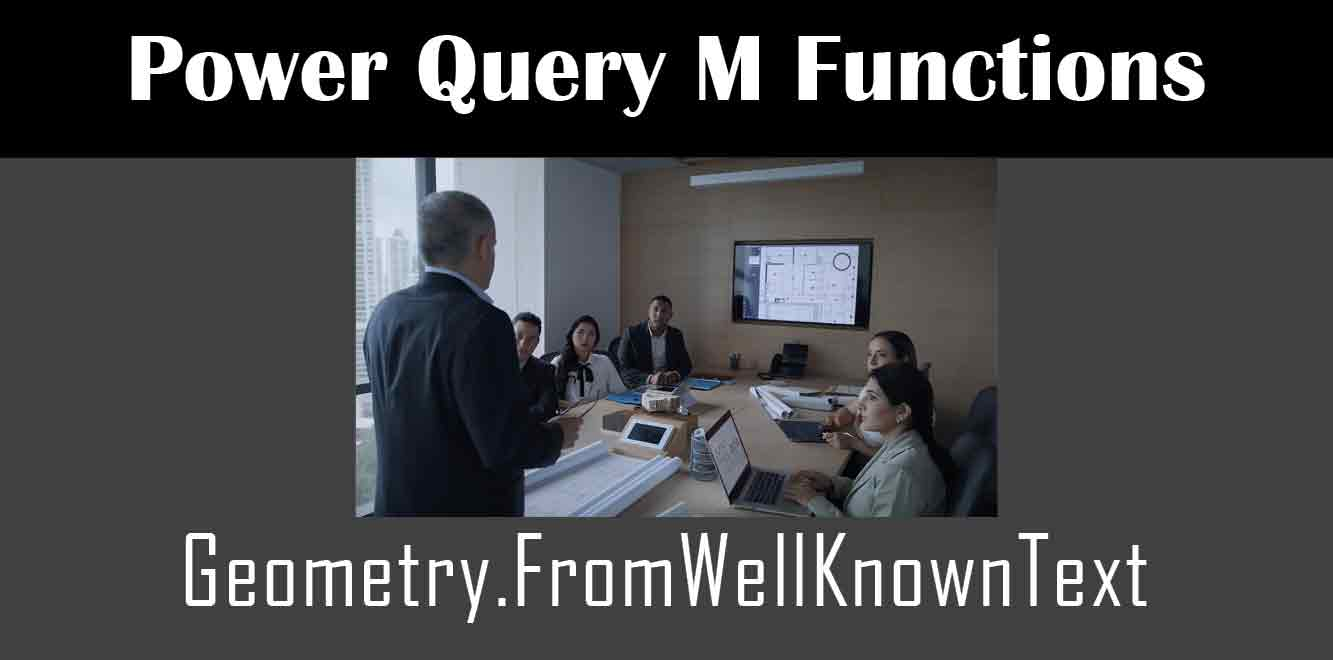# Geometry.FromWellKnownText

## #The M code behind the Geometry.FromWellKnownText function is responsible for parsing the WKT string and creating a spatial value that can be used in Power Query. In order to fully understand the M code behind this function, we need to first understand what WKT is and how it represents geometric objects.

## What is Well-Known Text?

Well-known text (WKT) is a text markup language that is used to represent geometric objects. WKT is a part of the Open Geospatial Consortium (OGC) Simple Features Specification, which defines a standard for representing spatial data. WKT is used to represent points, lines, polygons, and other geometric objects in a human-readable format.

Here is an example of a WKT string that represents a polygon:

POLYGON ((30 10, 40 40, 20 40, 10 20, 30 10))

``` In this example, the polygon is defined by a series of points that form a closed shape. Each point is represented by its x and y coordinates, separated by a space. The entire string is enclosed in parentheses and preceded by the keyword "POLYGON". How Does Geometry.FromWellKnownText Work? The Geometry.FromWellKnownText function takes a WKT string as its input and returns a spatial value that represents the geometric object. The M code behind this function is responsible for parsing the WKT string and creating a spatial value that can be used in Power Query. Here is an example of how to use the Geometry.FromWellKnownText function in Power Query: ```

let

wktString = “POINT (30 10)”,

spatialValue = Geometry.FromWellKnownText(wktString)

in

spatialValue

``` In this example, we are passing a WKT string that represents a point to the Geometry.FromWellKnownText function. The function returns a spatial value that can be used in Power Query. The M Code Behind Geometry.FromWellKnownText The M code behind the Geometry.FromWellKnownText function is responsible for parsing the WKT string and creating a spatial value that can be used in Power Query. The code is written in the M language, which is a functional programming language used by Power Query. Here is an example of the M code that powers the Geometry.FromWellKnownText function: ```

let

ParseWKT = (wktString as text) as any =>

let

wktTokens = Text.Split(wktString, ” “),

geometryType = wktTokens{0},

coordinates = List.Skip(wktTokens),

spatialValue = CreateSpatialValue(geometryType, coordinates)

in

spatialValue,

CreateSpatialValue = (geometryType as text, coordinates as list) as any =>

let

spatialValue = type [#”Spatial” = Geometry.Type],

geometry = if geometryType = “POINT” then CreatePoint(coordinates)

else if geometryType = “LINESTRING” then CreateLineString(coordinates)

else if geometryType = “POLYGON” then CreatePolygon(coordinates)

else if geometryType = “MULTIPOINT” then CreateMultiPoint(coordinates)

else if geometryType = “MULTILINESTRING” then CreateMultiLineString(coordinates)

else if geometryType = “MULTIPOLYGON” then CreateMultiPolygon(coordinates)

else if geometryType = “GEOMETRYCOLLECTION” then CreateGeometryCollection(coordinates)

else null

in

spatialValue{#”Spatial”} = geometry,

spatialValue,

CreatePoint = (coordinates as list) as any =>

let

x = Number.FromText(coordinates{0}),

y = Number.FromText(coordinates{1}),

point = type [#”x” = number, #”y” = number][x = x, y = y]

in

point,

CreateLineString = (coordinates as list) as any =>

let

points = List.Transform(coordinates, (point) => CreatePoint(Text.Split(point, ” “))),

lineString = type [#”Points” = List.Type(point)][Points = points]

in

lineString,

CreatePolygon = (coordinates as list) as any =>

let

ring = List.First(coordinates),

points = List.Transform(ring, (point) => CreatePoint(Text.Split(point, ” “))),

polygon = type [#”Ring” = List.Type(point)][Ring = points]

in

polygon,

CreateMultiPoint = (coordinates as list) as any =>

let

points = List.Transform(coordinates, (point) => CreatePoint(Text.Split(point, ” “))),

multiPoint = type [#”Points” = List.Type(point)][Points = points]

in

multiPoint,

CreateMultiLineString = (coordinates as list) as any =>

let

lineStrings = List.Transform(coordinates, (lineString) => CreateLineString(Text.Split(lineString, “, “))),

multiLineString = type [#”LineStrings” = List.Type(lineString)][LineStrings = lineStrings]

in

multiLineString,

CreateMultiPolygon = (coordinates as list) as any =>

let

polygons = List.Transform(coordinates, (polygon) => CreatePolygon(Text.Split(polygon, “, “))),

multiPolygon = type [#”Polygons” = List.Type(polygon)][Polygons = polygons]

in

multiPolygon,

CreateGeometryCollection = (coordinates as list) as any =>

let

geometries = List.Transform(coordinates, (geometry) => ParseWKT(geometry)),

geometryCollection = type [#”Geometries” = List.Type(geometry)][Geometries = geometries]

in

geometryCollection

in

ParseWKT

``` In this code, we define several functions that are used to parse the WKT string and create a spatial value. The main function is ParseWKT, which takes a WKT string as its input and returns a spatial value. The function first splits the WKT string into tokens, then extracts the geometry type and coordinates. It then calls the CreateSpatialValue function to create the spatial value. The CreateSpatialValue function takes the geometry type and coordinates as its input and creates a spatial value based on the type of geometry. For example, if the geometry type is "POINT", the function calls the CreatePoint function to create a point object. The CreatePoint, CreateLineString, CreatePolygon, CreateMultiPoint, CreateMultiLineString, CreateMultiPolygon, and CreateGeometryCollection functions are responsible for creating the actual geometric objects based on the coordinates. The Geometry.FromWellKnownText function is a powerful tool in Power Query that allows you to convert WKT strings into spatial values that can be used in your data transformations. The M code behind this function is responsible for parsing the WKT string and creating the spatial value. By understanding the M code behind this function, you can gain a deeper understanding of how Power Query works and how it can be used to transform and analyze data. Power Query and M Training Courses by G Com Solutions (0800 998 9248)Power Query and M Intensive Training Course £1,050.00 – £26,550.00 Select optionsContinue Loading DonePower Query and M Introduction £395.00 – £9,750.00 Select optionsContinue Loading DonePower Query and M Intermediate £395.00 – £9,750.00 Select optionsContinue Loading DonePower Query and M Advanced £395.00 – £9,750.00 Select optionsContinue Loading Done Upcoming Courses Contact Us Subject Your Name (required) Company/Organisation Email (required) Telephone Training Course(s) Power BI Intensive TrainingPower BI introduction Power BI IntermediatePower BI AdvancedDAXPower Query MPower BI CertificationPower BI AdministrationPower PlatformPower AutomatePower AppsOTHER Your Message Upload Example Document(s) (Zip multiple files) ```
``` ```
``` ```
``` ```
``` ```
``` ```